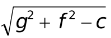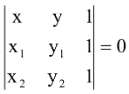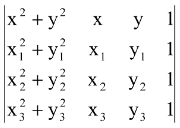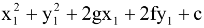# Introduction

## Certain Forms of the Equations of circles

(a) Centre (h, k) and radius r

The equation is (x – h)2 + (y - k)2 = r2  (1)

(b) General form of the equation of a circle is

x2 + y2 + 2gx + 2fy + c = 0 (2)

If we compare equation (1) and (2) we get the centre of (2) as (-g, -f) and radius =(c) Equation of a circle on the segment joining (x1, y1) and (x2, y2) as the ends of a diameter is given by (x – x1)(x – x2) + (y – y1)(y – y2) = 0 (3)

(d) General equation of a circle which passes through A(x1, y1) and B(x2, y2) may be written as

(x – x1)(x – x2) + (y – y1)( y – y2) + λ(4)

(e) The equation of the circle through 3 non-collinear points A(x1, y1), B(x2, y2) and C(x3, y3) is= 0

### Position of a Point

The point P(x1, y1) lies outside or inside the circle

S = x2 + y2 + 2gx + 2fy + c = 0 according as

S1 => or < 0